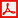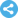International Journal of Mathematical Analysis and Applications
Manuscript Information

Feasible Length of Taylor Polynomial on Given Interval and Application to Find the Number of Roots of Equation
International Journal of Mathematical Analysis and Applications
Vol.1 , No. 5, Publication Date: Jan. 10, 2015, Page: 80-83
1575 Views Since January 10, 2015, 330 Downloads Since Apr. 12, 2015Paper in PDF (125K)Follow on

Authors

  Loc Nguyen, Institute of Mathematics, Hanoi, Vietnam.

Abstract

It is very necessary to represent arbitrary function as a polynomial in many situations because polynomial has many valuable properties. Fortunately, any analytic function can be approximated by Taylor polynomial. The higher the degree of Taylor polynomial is, the better the approximation is gained. There is problem that how to achieve optimal approximation with restriction that the degree is not so high because of computation cost. This research proposes a method to estimate feasible degree of Taylor polynomial so that it is likely that Taylor polynomial with degree being equal to or larger than such feasible degree is good approximation of a function in given interval. The feasible degree is called the feasible length of Taylor polynomial. The research also introduces an application that combines Sturm theorem and the method to approximate a function by Taylor polynomial with feasible length in order to count the number of roots of equation in given interval.

Keywords

Taylor Polynomial, Roots of Equation, Analytic Function Approximation, Feasible Length

Reference

  [Burdden 2011]. Burdden, R. L., Faires, J. D. Numerical Analysis, ninth edition. Brooks/Cole Cengage Learning Publisher Copyright 2011, 2005, 2001.  [Callahan 2008] James Callahan, J., Hoffman, K., Cox, D., O’Shea, D., Pollatsek, H., Senechal, L. Calculus in Context. The Five College Calculus Project Copyright 1994, 2008.  [Rosenberg 2006]. Rosenberg, J. The Integral Form of the Remainder in Taylor’s Theorem. Lectures notes in the course Math 141, Calculus II, Department of Mathematics, University of Maryland, 2006.  [Ta 2014]. Ta, D. P. Sturm’s theorem. Lectures notes in the course of Numerical Analysis, Vietnam Institute of Mathematics, 2014.  [Wikipedia 2014a]. Wikipedia. Taylor’s theorem. URL (last checked 30 August 2014) is http://en.wikipedia.org/wiki/Taylor%27s_theorem.  [Wikipedia 2014b]. Wikipedia. Stirling’s approximation. URL (last checked 30 August 2014) is http://en.wikipedia.org/wiki/Stirling%27s_approximation.  [Wikipedia 2014c]. Wikipedia. Sturm’s theorem. URL (last checked 30 August 2014) is http://en.wikipedia.org/wiki/Sturm%27s_theorem.